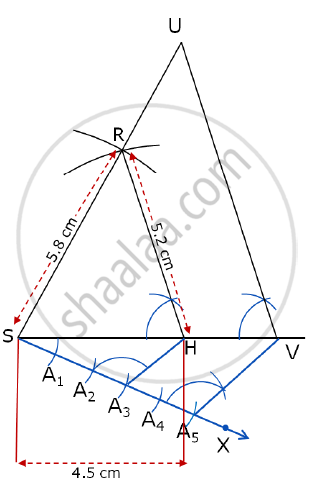# ΔSHR ~ ΔSVU. In ΔSHR, SH = 4.5 cm, HR = 5.2 cm, SR = 5.8 cm and SH/SV=3/5. Construct ΔSVU. - Geometry

Sum

ΔSHR ~ ΔSVU. In ΔSHR, SH = 4.5 cm, HR = 5.2 cm, SR = 5.8 cm and (SH)/(SV)=3/5. Construct ΔSVU.

#### Solution

Steps of construction:

1. Construct the Δ SHR with the given measurements. For this draw SH of length 4.5 cm.
2. Taking S as the centre and radius equal to 5.8 cm draw an arc above SH.
3. Taking H as the centre and radius equal to 5.2 cm draw an arc to intersect the previous arc. Name the point of intersection as R.
4. Join SR and HR. Δ SHR with the given measurements is constructed. Extend SH and SR further on the right side.
5. Draw any ray SX making an acute angle ( i.e; 45° ) with SH on the side opposite to the vertex R.
6. Locate 5 points. (the ratio of old triangle to new triangle is 3/5 and 5 > 3). Locate A1, A2, A3, A4 and A5 on AX so that SA1 = A1A2 = A2A3 = A3A4 = A4A5.
7. Join A3H and draw a line through A5 parallel to A3H, intersecting the extended part of SH at V.
8. Draw a line VU through V parallel to HR.

Δ SVU is the required triangle.Concept: Division of a Line Segment
Is there an error in this question or solution?